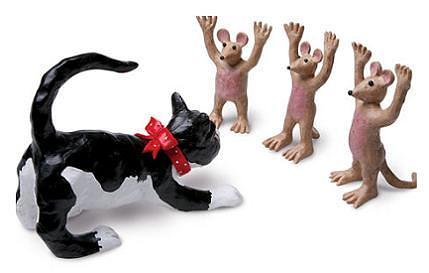# THE GAME OF CATS AND MICEA number of cats got together and decided to kill between them 999919 mice. Every cat killed an equal number of mice.
How many cats do you think there were?
Ob, by the way let me clarify just two points—it is not one cat killed the lot, because I have said ‘Cats’ and it is not-999919 cats each killed one mouse, because I have used the word ‘mice’.
I can give you just one clue—each cat killed more mice than there were cats.

There were 991 cats, and each of them killed 1009 mice.

To check, 991*1009 = 999919

Solution:

Get the prime factorization of 999919
The prime factors are 991 and 1009 only

Using fermat’s method find the Prime factor.
Now Let n = 999919 and square root is appx 1000
so i = 1000 , we find that sqrt(999^-999919)
= sqrt(81) = 9
Then 1000 – 9= 991 and 1000+ 9 = 1009
By multificaton we verify that 991*1009 = 999919
As Each cat killed more mice than the numebr of cats
Hence there were 991 cats and each cat killed 1009 mice.